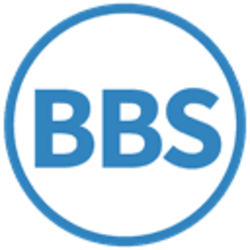# Carry (arithmetic)

Use Wikipedia with dynamical search help in all languages ...

Wikipedia - How to create a pageIn elementary arithmetic, a carry is a digit that is transferred from one column of digits to another column of more significant digits. It is part of the standard algorithm to add numbers together by starting with the rightmost digits and working to the left. For example, when 6 and 7 are added to make 13, the "3" is written to the same column and the "1" is carried to the left. When used in subtraction the operation is called a borrow.

Carrying is emphasized in traditional mathematics, while curricula based on reform mathematics do not emphasize any specific method to find a correct answer.[citation needed]

Carrying makes a few appearances in higher mathematics as well. In computing, carrying is an important function of adder circuits.

## Manual arithmetic

A typical example of carry is in the following pencil-and-paper addition:

```  1
27
+ 59
----
86
```

7 + 9 = 16, and the digit 1 is the carry.

The opposite is a borrow, as in

``` −1
47
− 19
----
28
```

Here, 7 − 9 = −2, so try (10 − 9) + 7 = 8, and the 10 is got by taking ("borrowing") 1 from the next digit to the left. There are two ways in which this is commonly taught:

1. The ten is moved from the next digit left, leaving in this example 3 − 1 in the tens column. According to this method, the term "borrow" is a misnomer, since the ten is never paid back.
2. The ten is copied from the next digit left, and then 'paid back' by adding it to the subtrahend in the column from which it was 'borrowed', giving in this example 4 − (1 + 1) in the tens column.

## Mathematics education

Traditionally, carry is taught in the addition of multi-digit numbers in the 2nd or late first year of elementary school. However, since the late 20th century, many widely adopted curricula developed in the United States such as TERC omitted instruction of the traditional carry method in favor of invented arithmetic methods, and methods using coloring, manipulatives, and charts. Such omissions were criticized by such groups as Mathematically Correct, and some states and districts have since abandoned this experiment, though it remains widely used.[citation needed]

## Higher mathematics

Kummer's theorem states that the number of carries involved in adding two numbers in base $p$is equal to the exponent of the highest power of $p$dividing a certain binomial coefficient.

When several random numbers of many digits are added, the statistics of the carry digits bears an unexpected connection with Eulerian numbers and the statistics of riffle shuffle permutations.

In abstract algebra, the carry operation for two-digit numbers can be formalized using the language of group cohomology. This viewpoint can be applied to alternative characterizations of the real numbers.

## Mechanical calculators

Carry represents one of the basic challenges facing designers and builders of mechanical calculators. They face two basic difficulties: The first one stems from the fact that a carry can require several digits to change: in order to add 1 to 999, the machine has to increment 4 different digits. Another challenge is the fact that the carry can "develop" before the next digit finished the addition operation.

Most mechanical calculators implement carry by executing a separate carry cycle after the addition itself. During the addition, each carry is "signaled" rather than performed, and during the carry cycle, the machine increments the digits above the "triggered" digits. This operation has to be performed sequentially, starting with the ones digit, then the tens, the hundreds, and so on, since adding the carry can generate a new carry in the next digit.

Some machines, notably Pascal's calculator, the second known calculator to be built, and the oldest surviving, use a different method: incrementing the digit from 0 to 9, cocks a mechanical device to store energy, and the next increment, which moves the digit from 9 to 0, releases this energy to increment the next digit by 1. Pascal used weights and gravity in his machine. Another notable machine using similar method is the highly successful 19th century Comptometer, which replaced the weights with springs.

Some innovative machines use continuous transmission: adding 1 to any digit, advances the next one by 1/10 (which in turn advances the next one by 1/100 and so on). Some innovative early calculators, notably Chebyshev calculator from 1870, and a design by Selling, from 1886, used this method, but neither were successful. In the early 1930, Marchant calculator implemented continuous transmission with great success, starting with the aptly named "Silent Speed" calculator. Marchant (later to become SCM Corporation) continued to use and improve it, and made continuous-transmission calculators with unmatched speed, into the late 1960s, to the end of the mechanical calculator era.

## Computing

When speaking of a digital circuit like an adder, the word carry is used in a similar sense.

In most computers, the carry from the most significant bit of an arithmetic operation (or bit shifted out from a shift operation) is placed in a special carry bit which can be used as a carry-in for multiple precision arithmetic or tested and used to control execution of a computer program. The same carry bit is also generally used to indicate borrows in subtraction, though the bit's meaning is inverted due to the effects of two's complement arithmetic. Normally, a carry bit value of "1" signifies that an addition overflowed the ALU, and must be accounted for when adding data words of lengths greater than that of the CPU. For subtractive operations, two (opposite) conventions are employed as most machines set the carry flag on borrow while some machines (such as the 6502 and the PIC) instead reset the carry flag on borrow (and vice versa).

## DuckDuckGoThis page is funded by cryptomining